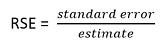# Precision Standards Guidelines for Reporting MEPS-IC Descriptive Statistics

The following statistical standards are applied for tabular estimates that can be obtained from the MEPS website and are recommended as general rules for researchers analyzing MEPS-IC data. All tables and table cells on the MEPS website (https://meps.ahrq.gov/mepsweb/data_stats/quick_tables.jsp) must satisfy U.S. Census Bureau disclosure review rules. These guidelines are based upon table cell size standards as well as relative standard error (RSE) standards, where the relative standard error of an estimate is defined as the standard error of the estimate divided by that estimate:## For all estimates except percentage estimates <5%

1. Estimates based on 20 or more observations (i.e., unweighted sample size of 20 or more) are published, but estimates with a relative standard error (RSE) of 30 percent or more are flagged with an * to indicate unreliable precision.

2. Estimates based on 10–19 observations are published only if the relative standard error is less than 20 percent.

3. Estimates based on 3–9 observations are published only if the relative standard error is less than 10 percent.

## For percentage estimates <5%

1. Estimates based on 3 or more observations are published only if the standard error is less than 2.5 percentage points (irrespective of RSE)1.

1 The purpose of this guideline is to avoid suppressing estimates of characteristics with low estimated prevalence (e.g., ≤5%) due to large RSEs attributable to small denominators. For example, the RSE for an estimate of 2% with an SE of 1 percentage point is .50. In this situation, the upper end of a 95% confidence interval would be around 4% which provides useful information that the prevalence of the characteristic is fairly uncommon (i.e. likely no higher than 4%). However, if the estimate had been 50% with an RSE of .50, then the 95% confidence interval would span from around 0% to 100%, which would clearly be too imprecise to provide any useful information.

Table Footnotes:
* Figure does not meet standard of reliability or precision (i.e., RSE ≥0.30).
-- Estimate suppressed due to small number of observations in cell or high standard error.